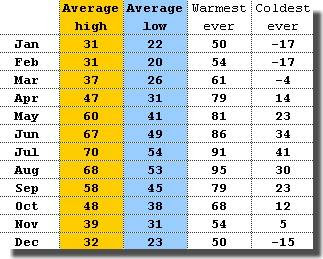fredag 9 september 2011

Climate Sensitivity from Basic Data < 0.4 CVariation of monthly mean value temperature in Stockholm over the year.

The basic model of global climate, used by e.g. Spencer and Lindzen to determine climate sensitivity, takes the form
• C dT/dt = F(t) - lambda T(t),
where T(t) is some zonal temperature over some period of time t, F(t) is forcing, C is a constant heat capacity and lambda is a positive constant. Here climate sensitivity S = 1/lambda measures the change of temperature per unit change of forcing. The model underlies the recent Spencer-Braswell paper causing an uproar among climate alarmists.

The model can be used to determine S by fitting the model to measurements of T(t) and F(t) over time. If S is small then climate is not sensitive to changes in forcing e.g. from increased CO2.

To understand the dependence of S on characteristics of data (amplitudes and phase lag), assume F(t) = cos(t) with corresponding solution
• T(t) = A (lambda cos (t) + C sin(t)), where A = 1/(C^2 + lambda^2).
We see that the phase lag is determined by the quotient lambda/C:
• small lambda/C: phase lag 1/4 period = large phase lag
• large lambda/C: small phase lag
• lambda/C = 1: phase lag 1/8 period.
Let us now consider two specific cases: (i) diurnal and (ii) yearly variation of some zonal temperature T(t) under varying insolation F(t).

Diurnal:
• variation of F(t) = 200 W/m2 (from day to night)
• variation of T(t) = 10 C
• phase lag = 3 hours (max temp at 3 pm)
• C = lambda > 10
Yearly:
• variation of F(t) = 150 W/m2 (from Summer to Winther)
• variation of T(t) = 15 C
• phase lage = 1.5 month (max temp mid August)
• lambda > C > 10.
In both cases we find a climate sensitivity S < 0.1. We find that the value S =1 by IPCC is a factor 10 too big.

There is massive data duplicating the above and there is thus massive data indicating that:
• the global warming from doubled CO2 of 3-4 C by IPCC, is a factor 10 too big.
Note that climate has a natural variability over day and night and over the year under large variations of the insolation-forcing, which can be used to assess climate sensitivity with respect to small CO2 forcing.

2 kommentarer:

1.Something is wrong with your Stockholm warmest ever and coolest ever temperatures particularly July. There is no way you could have 91C as the warmest. I think people would expire at that temperature. You should be able to get temperature data from KNMI Explorer.

2.Oh! Oh! Just thought the temperatures could be in degree F but that should not be for Stockholm. I am used to C or K.